# Linear Search in Array

## Array Linear Search in C

In this article, I am going to discuss Array Linear Search in C Language with Examples. Please read our previous article, where we discussed How to Delete an Element in an Array. In this article, we will see different methods for searching an element in an array. Basically, there are two methods for searching:Linear Search and Binary Search. There are other methods also but we will discuss those methods in our upcoming articles. Now, first, we will discuss linear search then in our next article, we will see binary search.

##### Linear Search in Array:

Let’s take an example of an array A .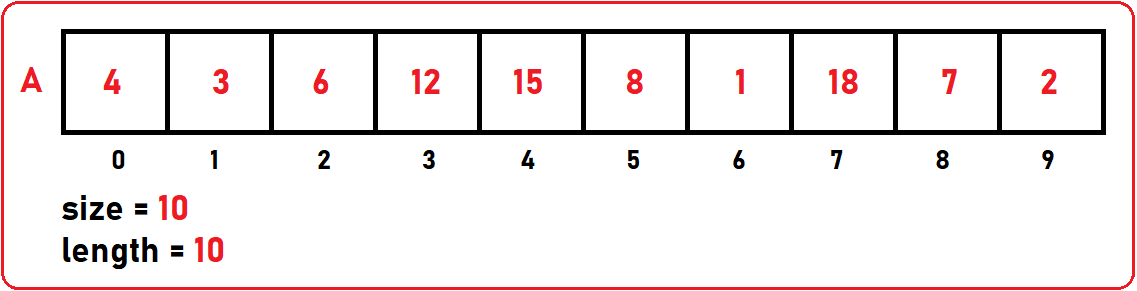We have created an array of name A (size = 10) and insert 10 (length = 10) elements inside this array. I have taken unique elements, which means there is no duplicate element in our array. If there are multiple copies then if we are searching, we may get any one of the copies, we may not be able to reach another copy of it.

In our array, if I want to search for any key (the value we are searching for usually call it a key) element. Let’s find where is key = 8. In the linear search method, we will check for the key in the array linearly by checking the elements one by one. So, key = 8 is stored at the index of 5. So, it is a successful search.

Let’s take another key which is 11. So, we will start comparing from index 0 to length-1, but this time we are unable to find the key 11. If we have reached the end of the list and the element is not found, then it will be known as an unsuccessful search.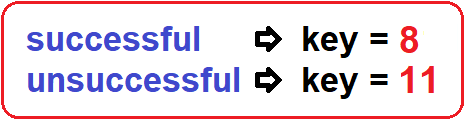The pseudo-code for the linear search is given below: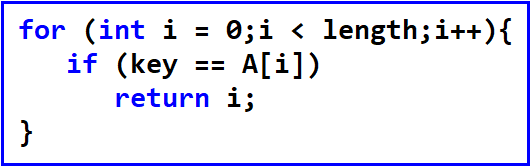##### Time Complexity:

It will take constant time (O (1)) if the key element is present at the index of 0. And it will take O(n) time when the key element is present at the index of length – 1.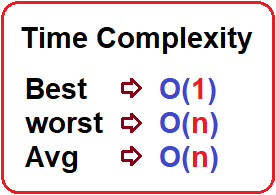Let’s have a look at the function code for linear search: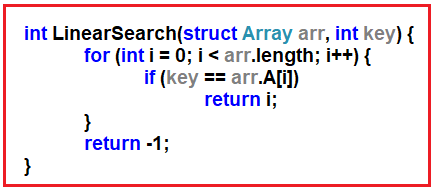##### Improved Linear Search:

In the Improved Linear Search, if we found that element in our array then we have to swap the element with the previous index in the given array so that for the next time when we will search the same element it will take less time for search that element.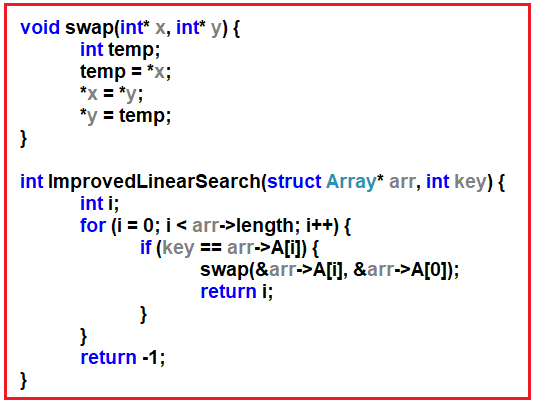##### Complete Linear Search Code in C Language:
```#include <stdio.h>
#include <stdlib.h>

struct Array {
int* A;
int size;
int length;
};

void swap(int* x, int* y) {
int temp;
temp = *x;
*x = *y;
*y = temp;
}

int LinearSearch(struct Array arr, int key) {
for (int i = 0; i < arr.length; i++) {
if (key == arr.A[i])
return i;
}
return -1;
}

int ImprovedLinearSearch(struct Array* arr, int key) {
int i;
for (i = 0; i < arr->length; i++) {
if (key == arr->A[i]) {
swap(&arr->A[i], &arr->A);
return i;
}
}
return -1;
}

int main() {
struct Array arr;
int keyElement;

printf("Enter Size of an Array: ");
scanf("%d", &arr.size);

arr.A = (int*)malloc(sizeof(int) * arr.size);
arr.length = 0;

printf("Enter Number of Elements: ");
scanf("%d", &arr.length);

printf("Enter All Elements: \n");
for (int i = 0; i < arr.length; i++) {
scanf("%d", &arr.A[i]);
}

printf("\nEnter the Element which you want to find in the Array: \n");
scanf("%d", &keyElement);

printf("\nElement found at Index: %d", ImprovedLinearSearch(&arr, keyElement));

getchar();
}
```
###### Output: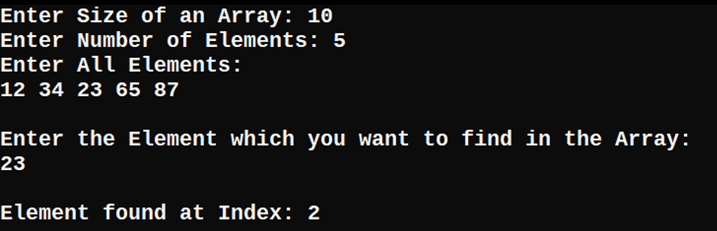In the next article, I am going to discuss Binary Search in Array with Examples. Here, in this article, I try to explain Array Linear Search in C Language with Examples and I hope you enjoy this Linear Search in Array with Examples article.